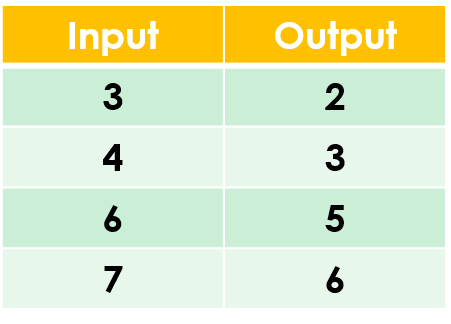1
visibility

Find the rule from the table below:• A

Subtract 1

• B

Subtract 3

The correct answer is "Subtract 1".

Please Note:
Subtraction is the operation that decreases a number by taking away from it.

Solution:
To find the correct rule, we consider the given table.

We observe that the given rule makes each number decrease. This implies subtraction.

We try subtracting 1 from each input number:

3 - 1 = 2
4 - 1 = 3
6 - 1 = 5
7 - 1 = 6

On subtracting 1 from the input numbers, we get the corresponding output numbers.

So, the rule is “subtract 1”.